Olympiad Test: Arithmetical Reasoning

# Olympiad Test: Arithmetical Reasoning

Test Description

## 15 Questions MCQ Test Science Olympiad Class 9 | Olympiad Test: Arithmetical Reasoning

Olympiad Test: Arithmetical Reasoning for Class 9 2022 is part of Science Olympiad Class 9 preparation. The Olympiad Test: Arithmetical Reasoning questions and answers have been prepared according to the Class 9 exam syllabus.The Olympiad Test: Arithmetical Reasoning MCQs are made for Class 9 2022 Exam. Find important definitions, questions, notes, meanings, examples, exercises, MCQs and online tests for Olympiad Test: Arithmetical Reasoning below.
Solutions of Olympiad Test: Arithmetical Reasoning questions in English are available as part of our Science Olympiad Class 9 for Class 9 & Olympiad Test: Arithmetical Reasoning solutions in Hindi for Science Olympiad Class 9 course. Download more important topics, notes, lectures and mock test series for Class 9 Exam by signing up for free. Attempt Olympiad Test: Arithmetical Reasoning | 15 questions in 15 minutes | Mock test for Class 9 preparation | Free important questions MCQ to study Science Olympiad Class 9 for Class 9 Exam | Download free PDF with solutions
 1 Crore+ students have signed up on EduRev. Have you?
Olympiad Test: Arithmetical Reasoning - Question 1

### Ram is three times as old as Mohan. Lokesh was twice as old as Ram four years ago. In four year’s time Ram will be 31. What is the difference between present ages of Mohan and Lokesh?

Detailed Solution for Olympiad Test: Arithmetical Reasoning - Question 1

Let Ram’s age = x, Mohan’s age = y, Lokesh’s age = z
∴ x = 3y …(1)
and z – 4 = 2 (x – 4)
z = 2x – 8 + 4 = 2x – 4 …(2)
x + 4 = 31 ⇒ x = 27
3y = 27 ⇒ y = 9
Using eq. (2) z = 2x – 4 = 2 × 27 – 4 = 50
Hence z – y = 50 – 9 = 41

Olympiad Test: Arithmetical Reasoning - Question 2

### Rajesh got twice as many sums wrong as he got right. If he attempted 84 sums in all. How many sums did he solve correctly?

Detailed Solution for Olympiad Test: Arithmetical Reasoning - Question 2

Let Rajesh solves x sums correctly
Right sums = x, wrong sums = 2x
∴ x + 2x = 84
⇒ 3x = 84
⇒ x = 28

Olympiad Test: Arithmetical Reasoning - Question 3

### Punam says, “If you reverse my own age, the figure represent my husband’s age. He is of course senior to me and the difference between our ages is one - eleventh of their sum. What is the age of Punam?

Detailed Solution for Olympiad Test: Arithmetical Reasoning - Question 3

Let Punam’s age be 10x + y
∴ Her husband’s age = 10y + x
Now
10y + x – 10x – y = 1/11 (10y + x + 10x + y)
⇒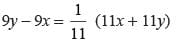⇒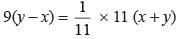⇒ 9y – 9x = x + y
⇒ 9y – y = x + 9x
⇒ 8y = 10x
⇒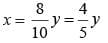If y = 5, then x = 4
Hence, Punam’s age = 10 × 4 + 5 = 45 years.

Olympiad Test: Arithmetical Reasoning - Question 4

A total of 324 coins of 20 paise & 25 paise make a sum of Rs. 71. What is the number of 25 paise coin?

Detailed Solution for Olympiad Test: Arithmetical Reasoning - Question 4

Let the number of 25 paise coins be x
No. of 20 paise coins = 324 – x
∴ 0.20 (324 – x) + 0.25 × x = 71
⇒ 64.8 – 0.20x + 0.25x = 71
⇒ 0.05x = 71 – 64.8
⇒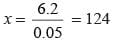Olympiad Test: Arithmetical Reasoning - Question 5

In a family, a couple has a son and a daughter. The age of the father is three times that of his daughter and the age of son is half that of his mother. The wife is 9 years younger than her husband and the brother is seven years older than his sister. What is the age of the mother?

Detailed Solution for Olympiad Test: Arithmetical Reasoning - Question 5

Let the daughter’s age be x
Father’s age = 3x
Mother’s age = 3x – 9
Son’s age = x + 7
∴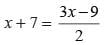⇒ 2x + 14 = 3x – 9 ⇒ x = 23
Mother’s age = 3x – 9 = 3 × 23 – 9 = 60 years

Olympiad Test: Arithmetical Reasoning - Question 6

In a group of cows and hens, the number of legs are 14 more than twice the number of heads. What is the number of cows?

Detailed Solution for Olympiad Test: Arithmetical Reasoning - Question 6

Let the number of cows be x & no. of hens be y
As per the question,
4x + 2y = 2 (x + y) + 14
⇒ 4x + 2y = 2x + 2y + 14
⇒ 2x = 14 ⇒ x = 7

Olympiad Test: Arithmetical Reasoning - Question 7

If few sweets, have to be distributed. If I keep 2, 3, or 4 in a pack, I am left with one sweet. If I keep 5 in a pack I am left with none. What is the minimum number of sweets I have to pack and distribute?

Detailed Solution for Olympiad Test: Arithmetical Reasoning - Question 7

If I keep 2 or 3 or 4 in a pack,
LCM of 2, 3, and 4 = 12
Then, the minimum number of sweets could be 12 + 1 = 13 so that 1 sweet left after packing.
But, if I keep 5 in a pack, I am left with none, (13 is not divisible by 5) then we have to take,
12 × 2 = 24
We have to add one so that 1 sweet left after packing in 2 or 3 or 4 groups and when in group of 5 then no sweets left. Therefore,
Total minimum sweets = 24 + 1 = 25 (25 is divisible by 5).
Hence, 25 is the correct answer.

Olympiad Test: Arithmetical Reasoning - Question 8

In a city 40% of adults are illiterate while 85% of the children are literate. If the ratio of the adults to that of the children is 2 : 3, what percent of the population is litereted.

Detailed Solution for Olympiad Test: Arithmetical Reasoning - Question 8

Let the no. of adults = 2x
No. of children = 3x
Literate population
= (100 – 40)% of 2x + 85% of 3x
= 60% of 2x + 85% of 3x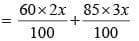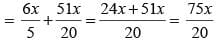Literate percent =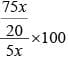Olympiad Test: Arithmetical Reasoning - Question 9

A says, “If B gives me Rs. 40, he well have half as much as C, but if C gives me Rs. 40, then three of us will all have the same amount. What is the total amount of money that A, B & C have between them?

Detailed Solution for Olympiad Test: Arithmetical Reasoning - Question 9

Given B – 40 =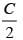⇒ 2B – 80 = C …(1)
and C – 40 = B …(2)
and C – 40 = A + 40 …(3)
From (1) & (2)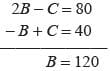C = B + 40 = 120 + 40 = 160
160 – 40 = A + 40 ⇒ A = 160 – 80 = 80
7 + B + C = 80 + 120 + 160 = 360

Olympiad Test: Arithmetical Reasoning - Question 10

Three bells ring after a regular interval of 36 seconds, 40 seconds and 48 seconds respectively. If the three bells start ringing at same time, then after how much time they will ring together?

Detailed Solution for Olympiad Test: Arithmetical Reasoning - Question 10

To find the time in which all the bells ring together, we need to find the LCM of 36, 40, 48
Prime factorization of 36 = 2 × 2 × 3 × 3
Prime factorization of 40 = 2 × 2 × 2 × 5
Prime factorization of 48 = 2 × 2 × 2 × 2 × 3
Hence, LCM of  36, 40, 48 = 2 × 2 × 2 × 2 × 3 × 3 × 5 = 720 seconds.
∴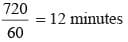Olympiad Test: Arithmetical Reasoning - Question 11

If a number is increased by 10% and then it is decreased by 10% then the value of number will

Detailed Solution for Olympiad Test: Arithmetical Reasoning - Question 11

Let the number be 100
100 + 10% of 100 = 100 + 10 = 110
and 110 – 10% of 110 = 100 –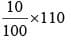= 110 – 11 = 99

Olympiad Test: Arithmetical Reasoning - Question 12

The cost price of 11 objects is Rs. 10 and the selling price of 10 objects is Rs. 11. What is the gain or loss percentage in whole transaction?

Detailed Solution for Olympiad Test: Arithmetical Reasoning - Question 12

Cost price of 11 objects = Rs. 10
Cost price of 1 object = Rs.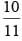Selling price of 10 objects = Rs. 11
Selling price of 1 object = Rs.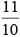∴ Gain percent =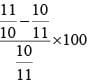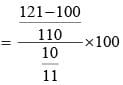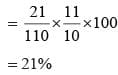Olympiad Test: Arithmetical Reasoning - Question 13

The total monthly income of 16 persons is Rs. 80800 and monthly income of one of the persons is greater than their average monthly income by 120%. What is the income of that person?

Detailed Solution for Olympiad Test: Arithmetical Reasoning - Question 13

Average monthly income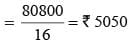Income of that person = 120% of 5050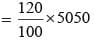= 12 × 505 = Rs. 6060

Olympiad Test: Arithmetical Reasoning - Question 14

On a certain festival, sweets were to be equally distributed among 200 students. But 50 students were absent, therefore each student get 4 sweets extra. What  is the number of sweets available for distribution?

Detailed Solution for Olympiad Test: Arithmetical Reasoning - Question 14

Total no. of students = 200
Let the no. of sweets = x
∴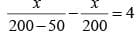⇒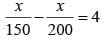⇒ 200x – 150x = 4 × 150 × 200
⇒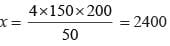Olympiad Test: Arithmetical Reasoning - Question 15

Three friends had lunch at a restaurant. Arjun paid 2/3 as much as Jay paid and Jay paid 1/2 as much as Rakesh paid. What fraction of the bill did Jay pay?

Detailed Solution for Olympiad Test: Arithmetical Reasoning - Question 15

Let Rakesh paid Rs. x.
Jay paid = x/2
Arjun paid =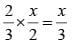∴ Total bill =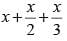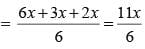Part of Jay =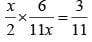## Science Olympiad Class 9

17 videos|6 docs|55 tests
 Use Code STAYHOME200 and get INR 200 additional OFF Use Coupon Code
Information about Olympiad Test: Arithmetical Reasoning Page
In this test you can find the Exam questions for Olympiad Test: Arithmetical Reasoning solved & explained in the simplest way possible. Besides giving Questions and answers for Olympiad Test: Arithmetical Reasoning, EduRev gives you an ample number of Online tests for practice

## Science Olympiad Class 9

17 videos|6 docs|55 tests